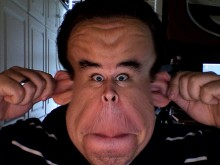# How To Make A Monkey Face In Text Messaging?@('_')@ is a good attempt at making the face of a monkey. In this face, you can see that the face is denoted with the '@' signs as the ears and the apostrophes as eyes, and the underscore as the mouth. Other animals can be made in the following ways: Enter letters and symbols to make a bird face. Key in "(*v*)" where each "*" is a bird eye and the "v" is the bird's beak. To create a gorilla face. Key in "8:]" where the "8" is the gorilla's eyes, the ":" is the nose and the "]" is the mouth. In order to create a cat face, key in "=^-^=" whereby each "=" is a cat whisker, each "^" is a cat ear and the "-" is the nose. For a dog face, key in ":o3" whereby each ":" is the dog's eyes, the lowercase "o" is the nose and the "3" is the dog's mouth. To make a pig face. Key in ":@)" when the ":" is the pig's eyes, the "@" is the pig's nose and the ")" is the pig's mouth. Try experimenting yourself with different types of animals and make sure you tell the world about your creations.

thanked the writer.
,,,,
@(o.o)@
(0)~
thanked the writer.
(\_/)
(o.o)
(___)0,, a bunny,,
d[-_-]b
(\_/)
(='.'=)
(")_(")
~~~~~~~:> snake
thanked the writer.
@(  ._.  )@  There. Fat monk
thanked the writer.
O(∩_∩)o
thanked the writer.
Anonymous commented
I think that both ears should be capitol.
O(o.o)o
(0)

yes
thanked the writer.

______
(_0_) (_0_)
------(00)

(______)

thanked the writer.
------m------m------
| |(o o)| |
||(~)||
thanked the writer.
(00)
(0)
thanked the writer.
0(o    o)0
(  T  )
( o )
thanked the writer.
C(o.o)3 part of its ear could be bitten off? LOL.
thanked the writer.
Anonymous commented
Why not just do 2 O's
@
8-0
@
top that
thanked the writer.
O_o ha its a weirded out face
thanked the writer.
^
(0)
thanked the writer.
[0.O] or [Q.Q]
get it?
thanked the writer.
(oYo)
(Y)
thanked the writer.
Smiley face what now
() ()
. ^ .
\__/

LOL its happy that all you get to use this now
thanked the writer.